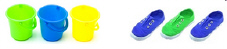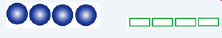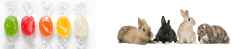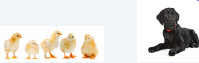KOA3.1
 Name:    KOA3.1

Multiple Choice
Identify the choice that best completes the statement or answers the question.

1.

Which picture problem will add up to 9?
 a.c.b.d.Multiple Response
Identify one or more choices that best complete the statement or answer the question.

2.

Mark any problem that will add up to 10.
 a. 2 + 8 c. 7 + 4 b. 6 + 3 d. 7 + 3

3.

Mark any problem that will add up to 5.
 a. 2 + 2 c. 4 + 1 b. 3 + 3 d. 1 + 4

4.

Mark any problem that will add up to 8.
 a. 4 + 4 c. 6 + 3 b. 5 + 3 d. 1 + 7